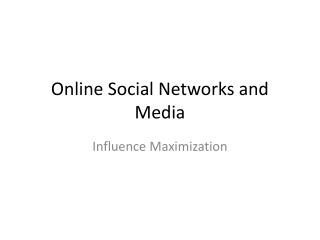# Online Social Networks and Media - PowerPoint PPT PresentationDownload PresentationOnline Social Networks and Media

Online Social Networks and Media
Download Presentation## Online Social Networks and Media

- - - - - - - - - - - - - - - - - - - - - - - - - - - E N D - - - - - - - - - - - - - - - - - - - - - - - - - - -
##### Presentation Transcript

1. Online Social Networks and Media Influence Maximization

2. Maximizing spread • Suppose that instead of a virus we have an item (product, idea, video) that propagates through contact • Word of mouth propagation. • An advertiser is interested in maximizing the spread of the item in the network • The holy grail of “viral marketing” • Question: which nodes should we “infect” so that we maximize the spread? [KKT2003]

3. Independent cascade model • Each node may be active (has the item) or inactive (does not have the item) • Time proceeds at discrete time-steps. At time t, every node v that became active in time t-1 actives a non-active neighbor w with probability puw. If it fails, it does not try again • The same as the simple SIR model

4. Influence maximization • Influence function: for a set of nodes A (target set) the influence s(A) is the expected number of active nodes at the end of the diffusion process if the item is originally placed in the nodes in A. • Influence maximization problem [KKT03]: Given an network, a diffusion model, and a value k, identify a set A of k nodes in the network that maximizess(A). • The problem is NP-hard

5. A Greedy algorithm • What is a simple algorithm for selecting the set A? • Computing s(A): perform multiple simulations of the process and take the average. • How good is the solution of this algorithm compared to the optimal solution? • Greedyalgorithm • Start with an empty set A • Proceed in k steps • At each step add the node u to the set A the maximizes the increase in function s(A) • The node that activates the most additional nodes

6. Approximation Algorithms • Suppose we have a (combinatorial) optimization problem, and X is an instance of the problem, OPT(X) is the value of the optimal solution for X, and ALG(X) is the value of the solution of an algorithm ALGfor X • In our case: X = (G,k) is the input instance, OPT(X) is the spread S(A*) of the optimal solution, GREEDY(X) is the spread S(A) of the solution of the Greedy algorithm • ALG is a good approximation algorithm if the ratio of OPT and ALG is bounded.

7. Approximation Ratio • For a maximization problem, the algorithm ALG is an -approximation algorithm, for , if for all input instances X, • The solution of ALG(X) has value at least α%that of the optimal • αis the approximation ratio of the algorithm • Ideally we would like αto be a small constant

8. Approximation Ratio for Influence Maximzation • The GREEDY algorithm has approximation ratio , for all X

9. Proof of approximation ratio • The spread function s has two properties: • s is monotone: • s is submodular: • The addition of node x to a set of nodes has greater effect (more activations) for a smaller set. • The diminishing returns property

10. Optimizing submodular functions • Theorem: A greedy algorithm that optimizes a monotone and submodularfunction s, each time adding to the solution A, the node x that maximizes the gain has approximation ratio • The spread of the Greedy solution is at least 63% that of the optimal

11. Submodularity of influence • Why is s(A)submodular? • How do we deal with the fact that influence is defined as an expectation? • We will use the fact that probabilistic propagation on a fixed graph can be viewed as deterministic propagation over a randomized graph • Express s(A) as an expectation over the input graphrather than the choices of the algorithm

12. Independent cascade model • Each edge (u,v) is considered only once, and it is “activated” with probability puv. • We can assume that all random choices have been made in advance • generate a sample subgraphof the input graph where edge (u,v) is included with probability puv • propagate the item deterministicallyon the input graph • the active nodes at the end of the process are the nodes reachable from the target set A • The influence function is obviously(?) submodular when propagation is deterministic • The weighted combination of submodular functions is also a submodular function

13. Linear threshold model • Again, each node may be activeor inactive • Every directed edge (v,u)in the graph has a weight bvu, such that • Each node u has a (randomly generated) threshold value Tu • Time proceeds in discrete time-steps. At time t an inactive node u becomes activeif • Related to the game-theoretic model of adoption.

14. Influence Maximization • KKT03 showed that in this case the influence s(A) is still a submodularfunction, using a similar technique • Assumes uniform random thresholds • The Greedy algorithm achieves a (1-1/e) approximation